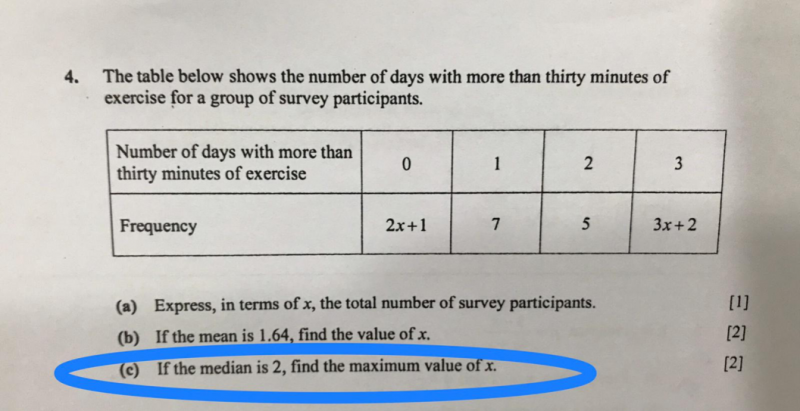# QuestionMedian is the middle number in a sorted list of numbers.

Hence if the median is 2 and to the maximum of x in value, below is how you should see

 0 1 2 2 3 2x+1 7 4 1 3x+2

∴ 2x +1 + 7 + 4 = 3x + 2

3x – 2x = 1+7+4-2

x = 10